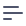# Joins

The `joins` parameter declares a block to define relationships between cubes. It allows users to access and compare fields from two or more cubes at the same time.

``````joins: {
TargetCubeName: {
relationship: `belongsTo` || `hasMany` || `hasOne`,
sql: `SQL ON clause`
}
}
``````

All joins are generated as `LEFT JOIN` and cube which defines a join serves as a main table and cube inside `joins` definition is one which goes to `LEFT JOIN` clause. Learn more about direction of joins here.

Semantics of `INNER JOIN` can be achieved with an additional filtering. For example by checking column value `IS NOT NULL` by using set filter.

## Parameters

### relationship

`relationship` enables you to describe the join relationship between joined cubes. It’s important to properly define the type of relationship in order for Cube.js to calculate accurate measures.

Note: It is very important to define the correct order of cubes in a join. It affects data in the result-set greatly.

The basic cube represents the left entity in a join, all others would be right. That means that all rows of the left cube are selected, while rows of the right depend on the condition.

For more information and specific examples, please take a look at our guides.

The three possible values for a relationship are:

#### hasOne

A `hasOne` relationship indicates a one-to-one connection with another cube. This relationship indicates that the one row in the cube can match only one row in the joined cube. For example, in a model containing users and user profiles, the users cube would have the following join:

``````cube("Users", {
joins: {
Profile: {
relationship: `hasOne`,
sql: `\${Users}.id = \${Profile}.user_id`
}
}
});
``````

#### hasMany

A `hasMany` relationship indicates a one-to-many connection with another cube. You'll often find this relationship on the "other side" of a `belongsTo` relationship. This relationship indicates that the one row in the cube can match many rows in the joined cube. For example, in a model containing authors and books, the authors cube would have the following join:

``````cube("Authors", {
joins: {
Books: {
relationship: `hasMany`,
sql: `\${Authors}.id = \${Books}.author_id`
}
}
});
``````

#### belongsTo

A `belongsTo` relationship indicates a many-to-one connection with another cube. You’ll often find this relationship on the “other side” of a `hasMany` relationship. This relationship indicates that the one row of the declaring cube matches a row in the joined instance, while the joined instance can have many rows in the declaring cube. For example, in a model containing orders and customers, the orders cube would have the following join:

``````cube("Orders", {
joins: {
Customers: {
relationship: `belongsTo`,
sql: `\${Orders}.customer_id = \${Customers}.id`
}
}
});
``````

### sql

`sql` is necessary to indicate a related column between cubes. It is important to properly specify a matching column when creating joins. Take a look at the example below:

``````  sql: `\${Orders}.customer_id = \${Customers}.id`
``````

## Setting a Primary Key

In order to make `join` work, it is necessary to define a `primaryKey` as specified below. It's required when a join is defined because Cube.js takes care of row multiplication issues.

Let's imagine you want to calculate `Order Amount` by `Order Item Product Name`. In this case, `Order` rows will be multiplied by the `Order Item` join due to the `hasMany` relationship. In order to produce correct results, Cube.js will select distinct primary keys from `Order` first and then will join these primary keys with `Order` to get the correct `Order Amount` sum result. Please note that `primaryKey` should be defined in the `dimensions` section.

``````dimensions: {
authorId: {
sql: `id`,
type: `number`,
primaryKey: true
}
}
``````

Note: Setting `primaryKey` to `true` will change the default value of the `shown` parameter to `false`. If you still want `shown` to be `true`—set it manually.

``````dimensions: {
authorId: {
sql: `id`,
type: `number`,
primaryKey: true,
shown: true
}
}
``````

If you don't have a single column in a cube's table that can act as a primary key, you can create a composite primary key as shown below.

The example uses Postgres string concatenation; note that SQL may be different depending on your database.

``````dimensions: {
id: {
sql: `\${CUBE}.user_id || '-' || \${CUBE}.signup_week || '-' || \${CUBE}.activity_week`,
type: `string`,
primaryKey: true
}
}
``````

## CUBE reference

When you have several joined cubes, you should accurately use columns’ names to avoid any mistakes. One way to make no mistakes is to use the `\${CUBE}` reference. It allows you to specify columns’ names in cubes without any ambiguity. During the implementation of the query, this reference will be used as an alias for a basic cube. Take a look at the following example:

``````dimensions: {
name: {
sql: `\${CUBE}.name`,
type: `string`
}
}
``````

## Transitive joins

Note

Join graph is directed and `A-B` join is different from `B-A`. Learn more about it here.

Cube.js automatically takes care of transitive joins. For example if you have following schema:

``````cube(`A`, {
// ...
joins: {
B: {
sql: `\${A}.b_id = \${B}.id`,
relationship: `belongsTo`
}
},

measures: {
count: {
type: `count`
}
}
});

cube(`B`, {
// ...
joins: {
C: {
sql: `\${B}.c_id = \${C}.id`,
relationship: `belongsTo`
}
}
});

cube(`C`, {
// ...

dimensions: {
category: {
sql: `category`,
type: `string`
}
}
});
``````

And following query:

``````{
measures: ['A.count'],
dimensions: ['C.category']
}
``````

Joins `A-B` and `B-C` will be resolved automatically. Cube.js uses Dijkstra algorithm to find join path between cubes given requested members.